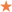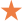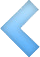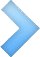138-2749-1088

商品全部分类
•❤ 收藏
• ## LEUCHTTURM1917德国灯塔A4+大开轻薄硬皮笔记本

300-500元

产品参数：

• 产品名称：LEUCHTTURM LEU-334917

• 品牌: LEUCHTTURM

• 型号: LEU-334917

• 风格: 人文 简约 复古 商务

• 颜色分类: 沙色 A4+(大开) 空白 沙色 A4+(大开) 点阵 沙色 A4+(大开) 横线 沙色 A4+(大开) 方格 红色 A4+(大开) 方格 红色 A4+(大开) 空白 红色 A4+(大开) 横线 红色 A4+（大开） 点阵 深灰色 A4+(大开) 方格 深灰色 A4+(大开) 点阵 深灰色 A4+(大开) 空白 深灰色 A4+(大开) 横线 桃粉色 A4+（大开） 空白 桃粉色 A4+（大开） 点阵 桃粉色 A4+（大开） 横线 桃粉色 A4+（大开） 方格 橙色 A4+（大开） 空白 橙色 A4+（大开） 点阵 橙色 A4+（大开） 横线 橙色 A4+（大开） 方格 军绿色 A4+（大开） 空白 军绿色 A4+（大开） 点阵 军绿色 A4+（大开） 横线 军绿色 A4+（大开） 方格 黑色 A4+（大开） 横格 黑色 A4+（大开） 点阵 黑色 A4+(大开) 空白 黑色 A4+（大开） 方格 北欧蓝 A4+(大开) 方格 北欧蓝 A4+(大开) 空白 北欧蓝 A4+(大开) 横线 北欧蓝 A4+(大开) 点阵 藏蓝色 A4+(大开) 横线 藏蓝色 A4+(大开) 空白 藏蓝色 A4+(大开) 方格 藏蓝色 A4+(大开) 点阵 太平洋绿 A4+(大开) 方格 太平洋绿 A4+（大开） 点阵 太平洋绿 A4+（大开） 空白 太平洋绿 A4+（大开） 横线 酒红色 A4+（大开） 点阵 酒红色 A4+(大开) 空白 酒红色 A4+(大开) 方格 酒红色 A4+（大开） 横线

• 页数: 100页以上

• 包装数量: 单本装

• 封面材质: 仿皮

• 生产企业: leuchtturm1917

• 封面硬度: 硬面抄

• 适用场景: 办公 送礼

• 幅面: 225*315mm

• 记事本分类: 通用笔记本

• 装订方式: 线装式装订

• 适用人群: 学生 白领

• 内页材质: 无酸纸

• ￥0.00
￥328.00
￥0.00
• 满意度：销量： 0

评论： 0 次

重量：0.00KG
• 数量：
• (库存99999)

• 产品名称：LEUCHTTURM LEU-334917

• 品牌: LEUCHTTURM

• 型号: LEU-334917

• 风格: 人文 简约 复古 商务

• 颜色分类: 沙色 A4+(大开) 空白 沙色 A4+(大开) 点阵 沙色 A4+(大开) 横线 沙色 A4+(大开) 方格 红色 A4+(大开) 方格 红色 A4+(大开) 空白 红色 A4+(大开) 横线 红色 A4+（大开） 点阵 深灰色 A4+(大开) 方格 深灰色 A4+(大开) 点阵 深灰色 A4+(大开) 空白 深灰色 A4+(大开) 横线 桃粉色 A4+（大开） 空白 桃粉色 A4+（大开） 点阵 桃粉色 A4+（大开） 横线 桃粉色 A4+（大开） 方格 橙色 A4+（大开） 空白 橙色 A4+（大开） 点阵 橙色 A4+（大开） 横线 橙色 A4+（大开） 方格 军绿色 A4+（大开） 空白 军绿色 A4+（大开） 点阵 军绿色 A4+（大开） 横线 军绿色 A4+（大开） 方格 黑色 A4+（大开） 横格 黑色 A4+（大开） 点阵 黑色 A4+(大开) 空白 黑色 A4+（大开） 方格 北欧蓝 A4+(大开) 方格 北欧蓝 A4+(大开) 空白 北欧蓝 A4+(大开) 横线 北欧蓝 A4+(大开) 点阵 藏蓝色 A4+(大开) 横线 藏蓝色 A4+(大开) 空白 藏蓝色 A4+(大开) 方格 藏蓝色 A4+(大开) 点阵 太平洋绿 A4+(大开) 方格 太平洋绿 A4+（大开） 点阵 太平洋绿 A4+（大开） 空白 太平洋绿 A4+（大开） 横线 酒红色 A4+（大开） 点阵 酒红色 A4+(大开) 空白 酒红色 A4+(大开) 方格 酒红色 A4+（大开） 横线

• 页数: 100页以上

• 包装数量: 单本装

• 封面材质: 仿皮

• 生产企业: leuchtturm1917

• 封面硬度: 硬面抄

• 适用场景: 办公 送礼

• 幅面: 225*315mm

• 记事本分类: 通用笔记本

• 装订方式: 线装式装订

• 适用人群: 学生 白领

• 内页材质: 无酸纸• 购买人 会员级别 数量 属性 购买时间
• 商品满意度 ：
•